# Manipulating List Data

Related tasks you can do in Excel 2007 include choosing rows at random from a list and displaying the unique values in a column in the worksheet (not in the down arrow list, which you can't normally work with). Generating a list of unique values in a column can give you important information, such as from which states you have customers or which categories of products sold in an hour.

Selecting rows randomly is useful for selecting customers to receive a special offer, deciding which days of the month to audit, or picking prize winners at an employee party. To choose rows, you can use the RAND function, which generates a random value between 0 and 1 and compares it with a test value included in the statement. A statement that returns a TRUE value 30 percent of the time would be RAND()<=30%; that is, whenever the random value was between 0 and .3, the result would be TRUE. You could use this statement to select each row in a list with a probability of 30 percent. A formula that displayed True when the value was equal to or less than 30 percent, and False otherwise, would be =IF(RAND()<=0.3,"True","False").

Tip

Because the RAND function is a volatile function (it recalculates its results every time you update the worksheet), you should copy the cells that contain the RAND function in a formula. Then, on the Home tab of the user interface, in the Clipboard group, click the Paste button down arrow and select Paste Values to replace the formula with its current result.

Excel 2007 has a new function, RANDBETWEEN, which enables you to generate a random value within a defined range. For example, the formula =RANDBETWEEN(1,100) would generate a random value from 1 to 100, inclusive.

The ability to focus on the data that's most vital to your current needs is important, but there are a few limitations. One limitation is that any formulas you create using the SUM and AVERAGE functions don't change their calculations if some of the rows used in the formula are hidden by the filter.

Excel 2007 provides two ways to find the total of a group of filtered cells. The first method is to use AutoCalculate. To use AutoCalculate, you select the cells you want to find the total for. When you do, Excel 2007 displays the average of values in the cells, the sum of the values in the cells, and the number of visible cells (count) in the selection. You'll find the display on the status bar at the lower edge of the Excel 2007 window.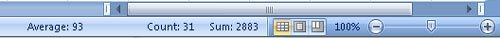When you use AutoCalculate, you aren't limited to finding the sum of the selected cells. To display the other functions you can use, right-click the AutoCalculate pane and select the function you want from the shortcut menu that appears.

AutoCalculate is great for finding a quick total or average for filtered cells, but it doesn't make the result available in the worksheet. Formulas such as =SUM(C3:C26) always consider every cell in the range, regardless of whether a cell's row is hidden by a filter, so you need to create a formula using the SUBTOTAL function. The SUBTOTAL function, which enables you to summarize only the visible cells in a range, has this syntax: SUBTOTAL(function_num, ref1, ref2, ...). The function_num argument holds the number of the operation you want to use to summarize your data. The ref1, ref2, and further arguments represent up to 29 ranges to include in the calculation. For example, the formula =SUBTOTAL(9, C3:C26, E3:E26, G3:G26) would find the sum of all values in the ranges C3:C26, E3:E26, and G3:G26.

Caution

Be sure to place your SUBTOTAL formula on a row that is even with or above the headers in the range you're filtering. If you don't, your filter might hide the formula's result!

The following table lists the summary operations available to you in a SUBTOTAL formula. The function numbers in the first column include all values in the summary; the function numbers in the second column summarize only those values that are visible within the worksheet.

Number (includes hidden values)

Number (ignores hidden values)

Function

Description

1

101

AVERAGE

Returns the average of the values in the range

2

102

COUNT

Counts the cells in the selected range that contain a number

3

103

COUNTA

Counts the nonblank cells in the selected range

4

104

MAX

Returns the largest (maximum) value in the selected range

5

105

MIN

Returns the smallest (minimum) value in the selected range

6

106

PRODUCT

Returns the result of multiplying all numbers in the selected range

7

107

STDEV

Calculates the standard deviation of values in the selected range by examining a sample of the values

8

108

STDEVP

Calculates the standard deviation of the values in the range by using all the values

9

109

SUM

Returns the result of adding all numbers in the range together

10

110

VAR

Calculates the variance of values in the selected range by examining a sample of the values

11

111

VARP

Calculates the variance of the values in the range by using all of the values

Note

Excel 2007 displays the available summary operations as part of the Formula AutoComplete functionality, so you don't need to remember the operation codes or look them up in the Help system.

If you want to find a list of the unique values in a data list row, click any cell in the data list, display the Data tab of the user interface, and then in the Sort & Filter group, click Advanced to display the Advanced Filter dialog box.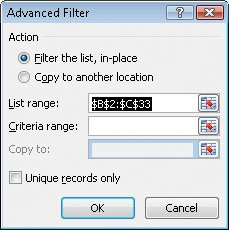In the List Range field, type the reference of the cell range you want to examine for unique values, select the Unique records only check box, and then click OK to have Excel 2007 display the first occurrence of each value in the column.

Important

Excel 2007 treats the first cell in the data range as a header cell, so it doesn't consider the cell as it builds the list of unique values. Be sure to include the header cell in your data range!

In this exercise, you will select random rows from a list of exceptions to identify package delivery misadventures to investigate, create a SUBTOTAL formula to summarize the visible cells in a filtered data list, and find the unique values in one column of a data list.

USE the ForFollowUp workbook in the practice file folder for this topic. This practice file is located in the My Documents\Microsoft Press\Excel SBS\Focusing folder.

OPEN the ForFollowUp workbook.

 1 Select cells G3:G27. The average of the values in the selected cells, the number of cells selected, and the total of the values in the selected cells appear in the AutoCalculate section of the status bar. 2 In cell K2, enter the formula =SUBTOTAL(101,G3:G27). The value \$15.76 appears in cell K2. 3 On the Data tab, in the Sort & Filter group, click Advanced. The Advanced AutoFilter dialog box appears. 4 In the List range field, type E2:E27. 5 Select the Unique records only check box, and then click OK. Excel 2007 displays the rows that contain the first occurrence of each different value in the selected range. Note Remember that you must include cell E2, the header cell, in the List range field range so that the filter doesn't display two occurrences of Northeast in the unique values list. To see what happens when you don't include the header cell, try changing the range in the List range field to E3:E27, selecting the Unique records only check box, and clicking OK.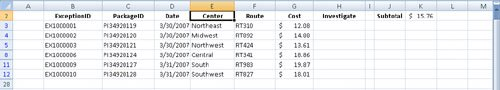6 On the Data tab, in the Sort & Filter group, click Clear. Excel 2007 removes the filter. 7 In cell H3, type the formula =IF(RAND()<0.15,"Yes","No") and press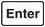. A value of Yes or No appears in cell H3, depending on the RAND function result. 8 Select cell H3 and then drag the fill handle down until it covers cell H27. Excel 2007 copies the formula into every cell in the range H3:H27. 9 With the range H3:H27 still selected, on the Home tab of the user interface, in the Clipboard group, click the Copy button. Excel 2007 copies the cell range's contents to the Clipboard. 10 Click the Paste button down arrow and then click Paste Values. Excel 2007 replaces the cells' formulas with the formulas' current results.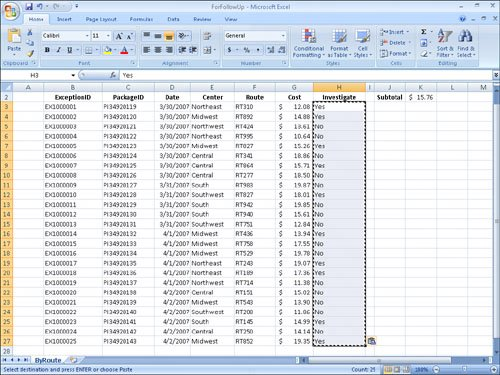CLOSE the ForFollowUp workbook.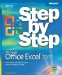MicrosoftВ® Office ExcelВ® 2007 Step by Step (Step By Step (Microsoft))
ISBN: 073562304X
EAN: 2147483647
Year: 2004
Pages: 143
Authors: Curtis Frye

Similar book on Amazon